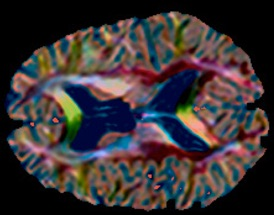PerceptVis project webpagePerceptVis is a method for perception-based visualization of manifold-valued medical images using distance preserving dimensionality reduction. Let’s break this definition down...

Visualization. PerceptVis is a visualization method. It takes as input “something complicated” and displays it in a simpler, more intuitive way. The input, in fact, is a medical image that is not easily and faithfully displayed with traditional approaches (e.g. as a grayscale image). The display produced by PerceptVis is a colour image, a format that humans are accustomed to looking at.

Manifold-valued medical images. These are the “complicated” images that we mentioned above. They are difficult to display because each of their pixels does not hold a single scalar (i.e. not a scalar-valued image), nor does it store an RGB vector of 3 numbers (not a vector-valued image). These images are more general than that because at every pixel there can be a general datum; each pixel could hold a a vector with, say, 30 elements, or even a matrix, e.g. a 3x3 array, or a more complicated tensor.  These type of images are collected every day at hospitals but, unfortunately, often the full information in these images is not fully utilized. For example, diffusion tensor magnetic resonance images (DTMRI) store a 2nd order, rank 3 positive semi-definite (PSD) tensor (or a 3x3 matrix) at each pixel, whereas a dynamic PET image stores a few dozens gamma activity samples (or a time activity curve) at each pixel, and so on. We consider the tensors, matrices, vectors, or scalars at image pixels to be samples from an underlying manifold, and hence the name manifold-valued images.

Perception. The main idea of PerceptVis is to visualize a manifold-valued medical image (or other manifold-valued data) as a color image, in a way that is faithful to the original image data. More precisely, the display should be such that (and this is the important part) similar manifold-valued pixels are displayed with perceptually similar colors. This requires the ability to 1) measure similarity between manifold-valued pixels, as well as 2) measure perceptual differences between colours. For the first, we resort to measuring distances on the manifold that these pixels belong to, or geodesic distances. The manifold maybe known analytically, e.g. the manifold of PSD matrices in the case of DTMRI, or maybe learned using manifold learning techniques. For the second, we work with a perceptually uniform 3D color space, CIELAB, in which the Euclidean distance between two colors in that space is proportional to perceived differences between those two colors.

Distance preserving dimensionality reduction. To realize the main objective: perceived color difference need to faithfully reflect manifold-valued pixel differences, we transform the high-dimensional pixel values to  3D CIELAB while ensuring that the pairwise geodesic distances between pixels remain unchanged after the transformation. This is generally not possible, so our approach finds the transformations that preserves these relative distances between high-D pixels as much as possible while (nonlinearly) projecting them down to 3D. For this we resort to the well-known algorithm for doing exactly that: Multi-Dimensional Scaling. A final step of scaling down or up all distances equally and rotating or translating all the points in 3D is performed to best utilize the CIELAB color gamut.

What is PerceptVis?

Brain

DTMRI